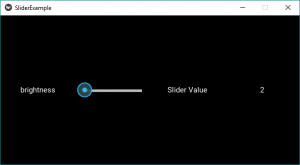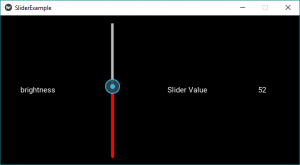# Python | Slider widget in Kivy

Kivy is a platform independent GUI tool in Python. As it can be run on Android, IOS, linux and Windows etc. It is basically used to develop the Android application, but it does not mean that it can not be used on Desktops applications.

Slider:
To work with the slider you first have to import the module which consists all featuers, functions of the slider i.e.

`Module: kivy.uix.slider`

The Slider widget looks like same we are using in android to increase the brightness, volume etc. It supports horizontal and vertical orientations, min/max values and a default value. Kivy supports several slider widget options for customizing the cursor, cursor image, border, background to be used in different orientations, region between the minimum value and the maximum value.
Kivy also supports to deal in term of Normalized value (range 0 to 1) rather than the real ranges supported by the slider.

Basic Approach to follow while creating Slider :

1) import kivy
2) import kivy App
3) import gridlayout(not compulsory according to need)
4) import Label(not compulsory according to need)
5) import Slider
6) import Numeric property
7) set minimum version(optional)
8) Extend the class
9) Add and return a widget
10) Run an instance of the class

Below is the code implementing slider:

 `# import kivy module  ` `import` `kivy  ` `   `  `# this restrict the kivy version i.e  ` `# below this kivy version you cannot  ` `# use the app or software  ` `kivy.require(``"1.9.1"``) ` ` `  `# Kivy Example App for the slider widget ` `from` `kivy.app ``import` `App ` ` `  `# The GridLayout arranges children in a matrix. ` `from` `kivy.uix.gridlayout ``import` `GridLayout ` ` `  `# If we will not import this module ` `# It will through the error ` `from` `kivy.uix.slider ``import` `Slider ` ` `  `# The Label widget is for rendering text. ` `from` `kivy.uix.label ``import` `Label ` ` `  `# Property that represents a numeric value ` `# within a minimum bound and / or maximum ` `# bound – within a numeric range. ` `from` `kivy.properties  ``import` `NumericProperty ` ` `  `# class in which we are defining the ` `# sliders and its effects ` `class` `WidgetContainer(GridLayout): ` ` `  `    ``def` `__init__(``self``, ``*``*``kwargs): ` `         `  `        ``# super function can be used to gain access  ` `        ``# to inherited methods from a parent or sibling  ` `        ``# class that has been overwritten in a class object.  ` `        ``super``(WidgetContainer, ``self``).__init__(``*``*``kwargs) ` ` `  `        ``# 4 columns in grid layout ` `        ``self``.cols ``=` `4` `         `  `        ``# declaring the slider and adding some effects to it ` `        ``self``.brightnessControl ``=` `Slider(``min` `=` `0``, ``max` `=` `100``) ` `          `  ` `  `        ``# 1st row - one label, one slider     ` `        ``self``.add_widget(Label(text ``=``'brightness'``)) ` `        ``self``.add_widget(``self``.brightnessControl)  ` ` `  `        ``# 2nd row - one label for caption, ` `        ``# one label for slider value ` `        ``self``.add_widget(Label(text ``=``'Slider Value'``)) ` `        ``self``.brightnessValue ``=` `Label(text ``=``'0'``) ` `        ``self``.add_widget(``self``.brightnessValue) ` ` `  ` `  `        ``# On the slider object Attach a callback ` `        ``# for the attribute named value ` `        ``self``.brightnessControl.bind(value ``=` `self``.on_value) ` `        `  `    ``# Adding functionality behind the slider ` `    ``# i.e when pressed increase the value ` `    ``def` `on_value(``self``, instance, brightness): ` `        ``self``.brightnessValue.text ``=` `"% d"``%` `brightness ` ` `  ` `  `# The app class ` `class` `SliderExample(App): ` `    ``def` `build(``self``): ` `        ``widgetContainer ``=` `WidgetContainer() ` `        ``return` `widgetContainer ` `  `  ` `  `# creating the object root for ButtonApp() class   ` `root ``=` `SliderExample() ` `   `  `# run function runs the whole program  ` `# i.e run() method which calls the  ` `# target function passed to the constructor.  ` `root.run()  `

Output:To add some styling and coloring to the slider, just replace the line no 42 with the below and add some new features also if you want. For text, styling uses the proper commands in text portion.

 `# declaring the slider and adding some effects to it ` `# By default its orientation is horizontal  ` `# if want to change to vertical do like below ` `        ``self``.brightnessControl ``=` `Slider(``min` `=` `0``, ``max` `=` `100``,  ` `                                        ``orientation ``=``'vertical'``, ` `                                        ``value_track ``=` `True``,  ` `                                        ``value_track_color ``=``[``1``, ``0``, ``0``, ``1``]) `

Output:Video to explain how slider works –

My Personal Notes arrow_drop_upCheck out this Author's contributed articles.

If you like GeeksforGeeks and would like to contribute, you can also write an article using contribute.geeksforgeeks.org or mail your article to contribute@geeksforgeeks.org. See your article appearing on the GeeksforGeeks main page and help other Geeks.

Please Improve this article if you find anything incorrect by clicking on the "Improve Article" button below.

Article Tags :

Be the First to upvote.

Please write to us at contribute@geeksforgeeks.org to report any issue with the above content.